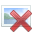# Basic Statistics Homework Question

Mod note: This post was moved from another forum section, so doesn't use the homework template.
A study of the amount of time it takes a mechanic to rebuild the transmission for a 2005 Chevrolet Cavalier shows that the mean is 8.4 hours and the standard deviation is 1.8 hours. If 40 mechanics are randomly selected, find the probability that their mean rebuild time exceeds 8.7 hours.

Is this the same as P(X-bar > 8.7) and P(Z > .16666666666..) because then the answer would be about 43% and the answers given are:

0.1469
0.1346
0.1946
0.1285

Can someone explain what I am doing wrong? Thanks in advance for your replies.

Last edited by a moderator:

Mark44
Mentor
Mod note: This post was moved from another forum section, so doesn't use the homework template.
A study of the amount of time it takes a mechanic to rebuild the transmission for a 2005 Chevrolet Cavalier shows that the mean is 8.4 hours and the standard deviation is 1.8 hours. If 40 mechanics are randomly selected, find the probability that their mean rebuild time exceeds 8.7 hours.

Is this the same as P(X-bar > 8.7) and P(Z > .16666666666..) because then the answer would be about 43% and the answers given are:

0.1469
0.1346
0.1946
0.1285

Can someone explain what I am doing wrong? Thanks in advance for your replies.
Please show your calculation for z. I'm pretty sure that's where the problem lies.

Please show your calculation for z. I'm pretty sure that's where the problem lies.

Mean= 8.4
x=8.7
SD=1.8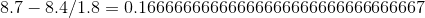Last edited by a moderator:
Mark44
Mentor
You have a sample of 40 mechanics. How does that need to fit into your calculations?

You have a sample of 40 mechanics. How does that need to fit into your calculations?
That is where I am stuck and unsure of what to do. Do I raise the probability of getting one mechanic whose time exceeds 8.7 to the power of 40?

So that would be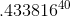?

Mark44
Mentor
No, that's not it. Your sample size (40) affects the standard deviation you use to calculate z. Your book should have this formula. As I recall, it's called the standard error of the mean, and represents the s.d. of all samples of size n.

BTW, I noticed that you also posted this question in the Precalc section. That's a no-no to post the same question in two or more forum sections.

No, that's not it. Your sample size (40) affects the standard deviation you use to calculate z. Your book should have this formula. As I recall, it's called the standard error of the mean, and represents the s.d. of all samples of size n.

BTW, I noticed that you also posted this question in the Precalc section. That's a no-no to post the same question in two or more forum sections.
I just found the equation in my book they dedicated such a little piece of text to it that I missed it when reading the first time. I calculated the standard error to of the mean to be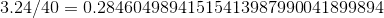Where do I go from this step?

I think you read it wrong. See http://en.wikipedia.org/wiki/Standard_error

Both my book and the Wikipedia link gives the same formula for calculating the error of the mean: Error of the mean= variance/sample size. When I do the calculations I get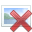. I don't see how I can apply that number to solving this problem. Is this the new standard deviation that I use to calculate the z-score?

^ Actually that's exactly what I needed to do haha. I don't quite understand why it works that way though. Can anyone explain it?

Mark44
Mentor
Both my book and the Wikipedia link gives the same formula for calculating the error of the mean: Error of the mean= variance/sample size. When I do the calculations I get.
In the Wiki link, it gives this: ##S.E. = \frac{s}{\sqrt{n}}##
Your calculation apparently doesn't include the square root.
xsgx said:
I don't see how I can apply that number to solving this problem. Is this the new standard deviation that I use to calculate the z-score?
You use it when you're looking at how samples of a given size are distributed.
From the Wiki page (emphasis added):
The standard error (SE) is the standard deviation of the sampling distribution of a statistic.

Ray Vickson